# Stable Isotope Mixing Models in R with simmr

## Introduction

tl;dr see the Quick Start vignette

`simmr` is a package designed to solve mixing equations for stable isotopic data within a Bayesian framework. This guide is designed to get researchers up and running with the package and giving them a full list of all the available features. No expertise is required in the use of R.

`simmr` is designed as an upgrade to the SIAR package and contains many of the same features. This new version contains a slightly more sophisticated mixing model, a simpler user interface, and more advanced plotting features. The key differences between SIAR and `simmr` are:

• `simmr` has a slightly richer mixing model based on code from the Parnell et al 2013 Environmetrics paper
• `simmr` does not have a menu system; all commands must be run through the console or script windows
• `simmr` uses ggplot2 to create graphs and JAGS to run the mixing model

We assume that you have a sound working knowledge of stable isotopic mixing models, and the assumptions and potential pitfalls associated with these models. A list of required reading is presented in Appendix A of this guide. We strongly recommend reading Philips et al 2015, Canadian Journal of Zoology for the basic assumptions and dos and don’ts of running mixing models.

We assume that if you have got this far you have installed R. We also recommend installing Rstudio as this provides a very neat interface to use R and `simmr`. The instructions below all assume you are using Rstudio.

If you find bugs in the software, or wish to suggest new features, please add your input to the `simmr` GitHub page.

## Installation of the `simmr` package

The `simmr` package uses the JAGS (Just Another Gibbs Sampler) programmer to run the stable isotope mixing model. Before you install simmr, visit the JAGS website and download and install JAGS for your operating system.

Next, start Rstudio and find the window with the command prompt (the symbol `>`). Type

``install.packages('simmr')``

It may ask you to pick your nearest CRAN mirror (the nearest site which hosts R packages). You will then see some activity on the screen as the `simmr` package and the other packages it uses are downloaded. The final line should then read:

`package 'simmr' successfully unpacked and MD5 sums checked`

You then need to load the package. Type

``library(simmr)``

This will load the `simmr` package and all the associated packages. You’ll need to type the `library(simmr)` command every time you start R. If you haven’t installed JAGS properly you will be informed at this point.

## Considerations before running simmr

Before getting started there are a couple of points to consider.

### Working with scripts

The best way to use the `simmr` package is by creating scripts. A script can be created in Rstudio by clicking `File > New File > Rscript`. This opens a text window which allows commands to be typed in order and saved. The command can be sent to the command prompt (which Rstudio calls the Console) by highlighting the command and clicking Run (or going to Code > Run Lines). There are also keyboard shortcuts to speed up the process. We strongly recommend you learn to run R via scripts.

### Data Structure

`simmr` can handle three different types of data structure:

• A single consumer. This may occur when you have only one data point on a single individual
• Multiple consumers. This may occur if you have multiple individuals in a single sampling period
• Multiple groups of consumers. This may occur if you have multiple consumers which are observed over different sampling periods/locations, different demographic groups, etc.

Unless you specify a grouping variable `simmr` assumes that all the observations are from the same group. If you have extra variables (e.g. explanatory variables) that you think may influence the dietary proportions, you should consider using (MixSIAR)[https://github.com/brianstock/MixSIAR] instead.

## Structure of the input files

The general structure for running `simmr` is as follows:

1. Call `simmr_load` on the data to get it into the right format
2. Plot the data in isotope space (‘iso-space’) using `plot`
3. Run the mixing model with `simmr_mcmc`
4. Explore the results with `plot` and `summary`
5. If you have multiple groups and want to compare output between them, use the `compare_groups’ function

For the next part of this document, we concentrate on simple examples without grouping structure.

### Step 1: Getting the data into simmr

`simmr` requires at minimum 3 input objects; the consumers or mixtures, the source means, and the source standard deviations. Optionally, you can also add correction data (also called trophic enrichment factors, TEFs) represented again as means and standard deviations, and concentration dependence values. The easiest way to get these objects into `simmr` is to create an Excel or similar file creating data and then copying it across as in the following example:

``````mix = matrix(c(-10.13, -10.72, -11.39, -11.18, -10.81, -10.7, -10.54,
-10.48, -9.93, -9.37, 11.59, 11.01, 10.59, 10.97, 11.52, 11.89,
11.73, 10.89, 11.05, 12.3), ncol = 2, nrow = 10)
colnames(mix) = c('d13C','d15N')
s_names = c("Zostera", "Grass", "U.lactuca", "Enteromorpha")
s_means = matrix(c(-14, -15.1, -11.03, -14.44, 3.06, 7.05, 13.72, 5.96), ncol = 2, nrow = 4)
s_sds = matrix(c(0.48, 0.38, 0.48, 0.43, 0.46, 0.39, 0.42, 0.48), ncol = 2, nrow = 4)
c_means = matrix(c(2.63, 1.59, 3.41, 3.04, 3.28, 2.34, 2.14, 2.36), ncol = 2, nrow = 4)
c_sds = matrix(c(0.41, 0.44, 0.34, 0.46, 0.46, 0.48, 0.46, 0.66), ncol = 2, nrow = 4)
conc = matrix(c(0.02, 0.1, 0.12, 0.04, 0.02, 0.1, 0.09, 0.05), ncol = 2, nrow = 4)``````

The `mix` object above contains the stable isotopic data for the consumers. The data should be listed as the consumer values for the first isotope, followed by the consumer values for the second isotope and so on. The `matrix` function turns this into a matrix (a rectangle of numbers) with 2 columns. The first column contains the data for isotope 1, and the second the data for isotope 2. Any number of isotopes and observations can be used. It is recommended but not necessary to give the mixtures column names representing the isotopes to which each column corresponds.

The source names are provided in the `s_names` object, and the source means and standard deviations in `s_means` and `s_sds`. These latter objects must also be matrices, where the number of rows is the number of sources, and the number of columns the number of isotopes. In each case, the data are included by listing the values for the first isotope, then the second isotope, and so on.

The correction data is stored in `c_means` and `c_sds`. Again this should be a matrix of the same dimension as `s_means` and `s_sds`. Finally the concentration dependencies (i.e. the elemental concentration values) are included as `conc`.

Some data sets are also included in `simmr` for quick access to examples. See `data(package = "simmr")` for the list. They can all be accessed via, e.g. `data("geese_data")`.

To load the data into simmr, use:

``````simmr_in = simmr_load(mixtures = mix,
source_names = s_names,
source_means = s_means,
source_sds = s_sds,
correction_means = c_means,
correction_sds = c_sds,
concentration_means = conc)``````

Remember that the `correction_means`, `correction_sds`, and `concentration_means` are optional.

### Step 2: Plotting the data in iso-space

We can now plot the raw isotopic data with:

``plot(simmr_in)``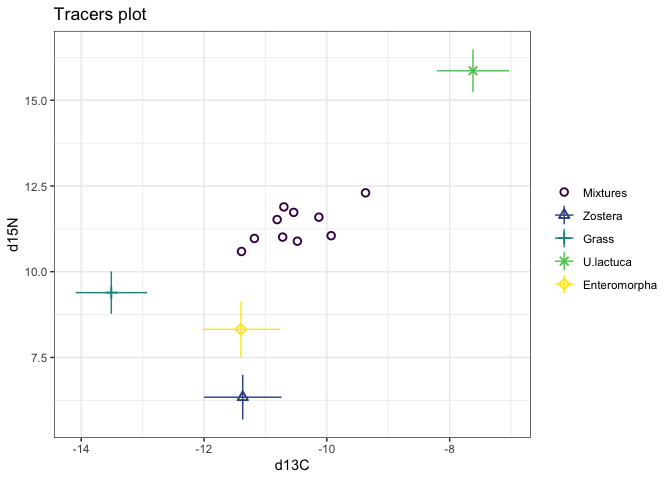This will produce a biplot with the isotope that is in the first column on the x-axis, and the isotope in the second column on the y-axis. You can make the plot slightly nicer with some extra arguments:

``````plot(simmr_in,
xlab = expression(paste(delta^13, "C (\u2030)",
sep = "")),
ylab = expression(paste(delta^15, "N (\u2030)",
sep = "")),
title = 'Isospace plot of example data')``````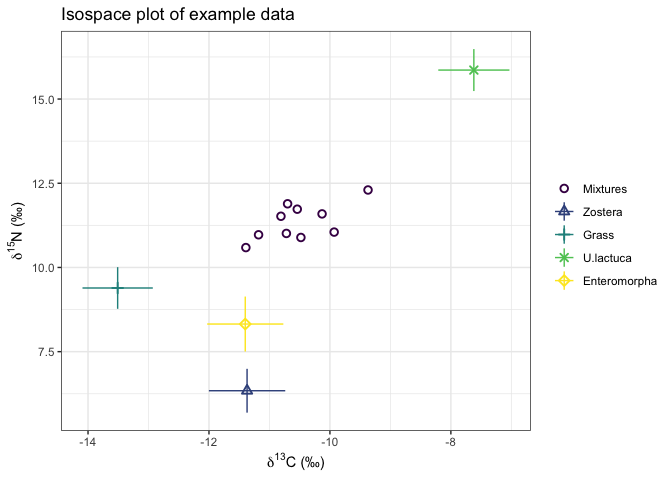See the help file `help(plot.simmr_input)` for more options on the plotting commands, including the ability to plot different tracers/isotopes when there are more than 2 isotopes.

If all the mixtures lie inside the mixing polygon defined by the sources, then the data are acceptable for running `simmr`. See Philips et al 2015, Canadian Journal of Zoology for more details on when data are suitable for running through a mixing model.

### Step 3: Running simmr

The next step is to actually run the model. This is achieved with the command:

``simmr_out = simmr_mcmc(simmr_in)``

This command takes the object `simmr_in` we created earlier and uses it as input for the model. It tells `simmr` to store the output from the model run in an object called `simmr_out`.

The model should take less than a minute to run, though this will depend on the speed of the computer you are using. Other data sets might take slightly longer or shorter depending on the number of sources, isotopes, and observations. The progress of the model is displayed on the command line window, which shows the percentage complete.

### Step 4: Checking the algorithm converged

Markov chain Monte Carlo (MCMC) works by repeatedly guessing the values of the dietary proportions and find those values which fit the data best. The initial guesses are usually poor and are discarded as part of an initial phase known as the burn-in. Subsequent iterations are then stored and used for the posterior distribution; the best estimates of the dietary proportions given the data and the model. Because it can take many thousands of iterations to move away from the initial guesses, convergence diagnostics can be created to check the model has run properly. In `simmr` this is done with:

``summary(simmr_out, type = 'diagnostics')``
``````##
## Summary for 1
## Gelman diagnostics - these values should all be close to 1.
## If not, try a longer run of simmr_mcmc.
##     deviance      Zostera        Grass    U.lactuca Enteromorpha     sd[d13C]
##            1            1            1            1            1            1
##     sd[d15N]
##            1``````

If the model run has converged properly the values should be close to 1. If they are above 1.1, we recommend a longer run. See `help(simmr_mcmc)` for how to do this.

### Step 5: Checking the model fit

You can check the fit of the model with a posterior predictive check. This is similar to a fitted values plot in a linear regression. If the data points (denoted by the plot as \(y\)) broadly lie in the fitted value intervals (denoted \(y_rep\); the default is a 50% interval) then the model is fitting well:

``post_pred = posterior_predictive(simmr_out)``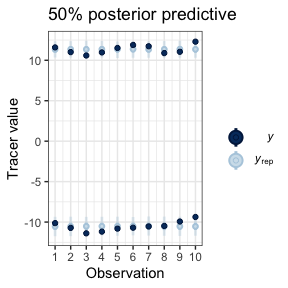``print(post_pred)``

The output includes a table showing which observations lie outside the posterior predictive and the proportion doing so, which should approximately match the proportion specified in the `posterior_predictive` function (default 50%).

### Step 6: Exploring the results

All SIMMs use informative (usually generalist) prior distributions as a default. You can plot the priors and the posteriors with:

``prior_viz(simmr_out)``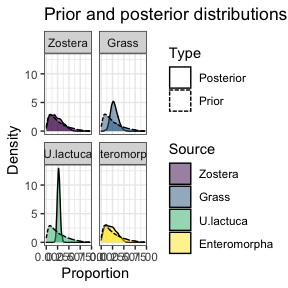`simmr` produces both textual and graphical summaries of the model run. Starting with the textual summaries, we can get tables of the means, standard deviations and credible intervals (the Bayesian equivalent of a confidence interval) with:

``summary(simmr_out, type = 'statistics')``
``````##
## Summary for 1
##                mean    sd
## deviance     36.324 3.219
## Zostera       0.221 0.131
## Grass         0.288 0.080
## U.lactuca     0.275 0.032
## Enteromorpha  0.216 0.120
## sd[d13C]      0.557 0.243
## sd[d15N]      0.430 0.230``````
``summary(simmr_out, type = 'quantiles')``
``````##
## Summary for 1
##                2.5%    25%    50%    75%  97.5%
## deviance     32.231 33.925 35.676 37.964 44.025
## Zostera       0.030  0.112  0.200  0.314  0.503
## Grass         0.148  0.232  0.281  0.338  0.459
## U.lactuca     0.217  0.253  0.274  0.295  0.345
## Enteromorpha  0.033  0.115  0.204  0.302  0.461
## sd[d13C]      0.174  0.397  0.526  0.680  1.110
## sd[d15N]      0.055  0.275  0.405  0.551  0.950``````

These suggest that the dietary proportions for this model are quite uncertain. However we can see that the credible interval for U.lactuca is the narrowest, running from approximately 20% to 35% of the diet. The reason this one is the narrowest can be seen from the isospace plot - this source is the most clearly separated from the others.

`simmr` can also produce histograms, boxplots, density plots, and matrix plots of the output. Starting with the density plot:

``plot(simmr_out, type = 'density')``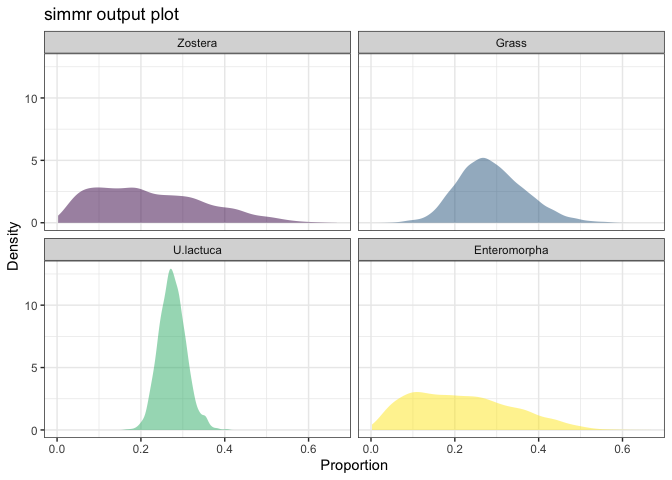We can see that Zostera and Enteromorpha are poorly constrained in comparison to Grass and especially U.lactuca. Again this is unsurprising since the isospace plot indicated that these were the two most clearly separated sources.

The most useful output plot is the matrix plot:

``plot(simmr_out, type = 'matrix')``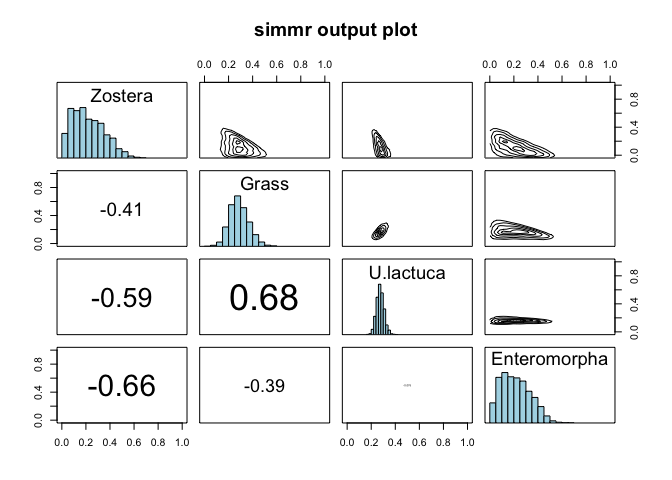This shows the source histograms on the diagonal, contour plots of the relationship between the sources on the upper diagonal, and the correlation between the sources on the lower diagonal. Large negative correlations indicate that the model cannot discern between the two sources; they may lie close together in iso-space. Large positive correlations are also possible when mixture data lie in a polygon consisting of multiple competing sources. Here the largest negative correlation is between Zostera and Enteromorpha. This is because they lie closest together in isospace. In general, high correlations (negative or positive) are indicative of the model being unable to determine which food sources are being consumed, and are an unavoidable part of stable isotope mixing models.

If you want to compare the dietary proportions between two different sources, you can use the `compare_sources` function. This takes two or more sources and compares the dietary proportions with an optional plot. For example:

``````compare_sources(simmr_out,
source_names = c('Zostera','U.lactuca'))``````
``## Prob ( proportion of Zostera > proportion of U.lactuca ) = 0.349``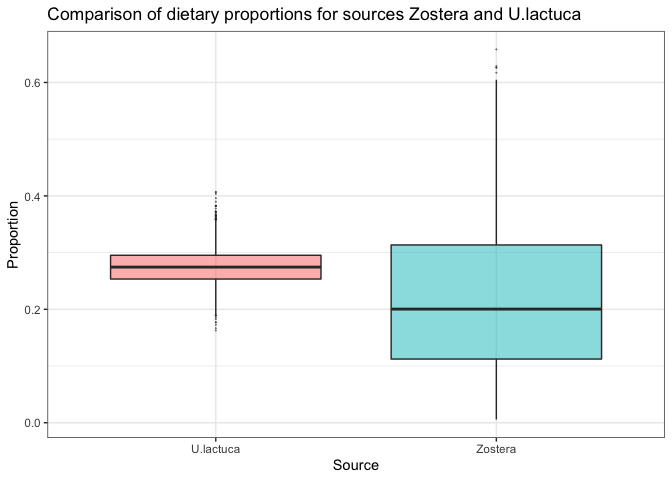This produces a direct probability that the dietary proportion for the first source is bigger than that of the second. If you want to compare more than two sources, specify them with:

``````compare_sources(simmr_out,
source_names = c('Zostera',
'U.lactuca',
'Enteromorpha'))``````
``````## Most popular orderings are as follows:
##                                    Probability
## Zostera > U.lactuca > Enteromorpha      0.3111
## Enteromorpha > U.lactuca > Zostera      0.2697
## U.lactuca > Enteromorpha > Zostera      0.2167
## U.lactuca > Zostera > Enteromorpha      0.1647
## Zostera > Enteromorpha > U.lactuca      0.0217
## Enteromorpha > Zostera > U.lactuca      0.0161``````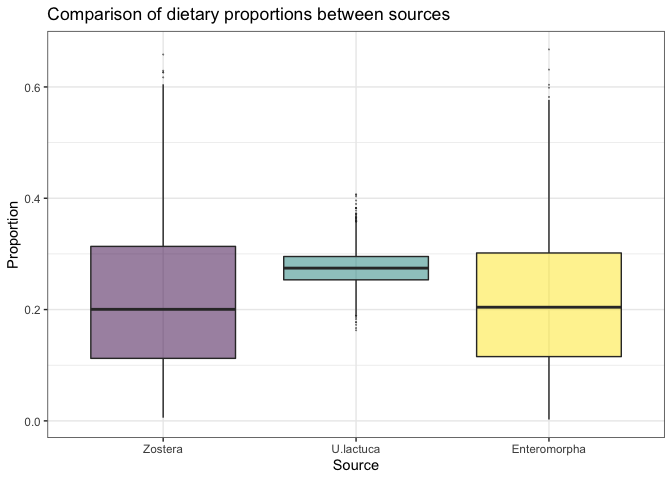For further information and options on comparing sources, see `help(compare_sources)`.

## Running `simmr` on multiple groups

In many cases we have data from different sampling locations, or different types of individuals (e.g. male/female) and we to compare between these groups. `simmr` can handle these data sets provided they all share the same sources, corrections and concentration dependence values.

A useful data set is given by Inger et al 2006 and provided as part of the original SIAR package. These data concern Brent Geese observed on 8 separate sampling periods.

These data are included in the package, and we can load these data into R with:

``data(geese_data)``

…and into simmr with:

``````simmr_groups = with(geese_data,
source_names = source_names,
source_means = source_means,
source_sds = source_sds,
correction_means = correction_means,
correction_sds = correction_sds,
concentration_means = concentration_means,
group = as.factor(paste('period',
mixtures[,3]))))``````

Note that the `group` object above is specified to be a factor but can also be an integer, the levels of which will appear in plots. However, when specifying groups in later commands you should use the integer values to reference which groups to plot

Next it is a matter of following the `simmr` commands as before to load in, with an extra argument specifying the groups:

When we create the isospace plot we can specify which groups we wish to plot:

``````plot(simmr_groups,
group = 1:8,
xlab = expression(paste(delta^13, "C (\u2030)",
sep = "")),
ylab = expression(paste(delta^15, "N (\u2030)",
sep = "")),
title = 'Isospace plot of Inger et al Geese data',
mix_name = 'Geese')``````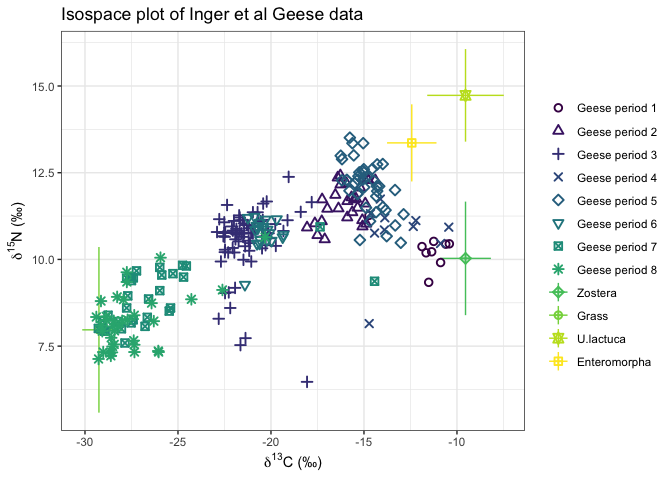In the above code `group = 1:8` can be changed specify any of the available groups. For example `group = 2` would plot just sampling period 2, or `group = c(1,3:7)` would plot just sampling period 1 and 3 to 7.

The command for running the `simmr` model is identical to before:

``simmr_groups_out = simmr_mcmc(simmr_groups)``

`simmr` will automatically run the model for each group in turn. This may take slightly longer than a standard single group run.

The `summary` command works the same as before. By default they will produce output for all groups, or you can specify the groups individually, e.g.:

``````summary(simmr_groups_out,
type = 'quantiles',
group = 1)``````
``````##
## Summary for period 1
##                2.5%    25%    50%    75%  97.5%
## deviance     51.083 52.515 53.990 56.250 62.976
## Zostera       0.376  0.520  0.594  0.669  0.799
## Grass         0.033  0.058  0.072  0.087  0.118
## U.lactuca     0.024  0.080  0.130  0.197  0.322
## Enteromorpha  0.022  0.091  0.169  0.267  0.485
## sd[]          0.070  0.532  0.889  1.329  2.511
## sd[]          0.015  0.167  0.355  0.618  1.390``````
``````summary(simmr_groups_out,
type = 'quantiles',
group = c(1,3))``````
``````##
## Summary for period 1
##                2.5%    25%    50%    75%  97.5%
## deviance     51.083 52.515 53.990 56.250 62.976
## Zostera       0.376  0.520  0.594  0.669  0.799
## Grass         0.033  0.058  0.072  0.087  0.118
## U.lactuca     0.024  0.080  0.130  0.197  0.322
## Enteromorpha  0.022  0.091  0.169  0.267  0.485
## sd[]          0.070  0.532  0.889  1.329  2.511
## sd[]          0.015  0.167  0.355  0.618  1.390
##
## Summary for period 3
##                 2.5%     25%     50%     75%   97.5%
## deviance     454.591 456.316 457.938 460.161 465.854
## Zostera        0.009   0.024   0.036   0.051   0.085
## Grass          0.391   0.418   0.428   0.437   0.452
## U.lactuca      0.292   0.398   0.443   0.478   0.526
## Enteromorpha   0.014   0.047   0.084   0.139   0.281
## sd[]           0.406   0.648   0.761   0.870   1.074
## sd[]           0.008   0.074   0.158   0.270   0.538``````
``````summary(simmr_groups_out,
type = c('quantiles','statistics'),
group = c(1,3))``````
``````##
## Summary for period 1
##                2.5%    25%    50%    75%  97.5%
## deviance     51.083 52.515 53.990 56.250 62.976
## Zostera       0.376  0.520  0.594  0.669  0.799
## Grass         0.033  0.058  0.072  0.087  0.118
## U.lactuca     0.024  0.080  0.130  0.197  0.322
## Enteromorpha  0.022  0.091  0.169  0.267  0.485
## sd[]          0.070  0.532  0.889  1.329  2.511
## sd[]          0.015  0.167  0.355  0.618  1.390
##                mean    sd
## deviance     54.805 3.163
## Zostera       0.593 0.108
## Grass         0.073 0.022
## U.lactuca     0.143 0.081
## Enteromorpha  0.192 0.126
## sd[]          0.984 0.654
## sd[]          0.443 0.372
##
## Summary for period 3
##                 2.5%     25%     50%     75%   97.5%
## deviance     454.591 456.316 457.938 460.161 465.854
## Zostera        0.009   0.024   0.036   0.051   0.085
## Grass          0.391   0.418   0.428   0.437   0.452
## U.lactuca      0.292   0.398   0.443   0.478   0.526
## Enteromorpha   0.014   0.047   0.084   0.139   0.281
## sd[]           0.406   0.648   0.761   0.870   1.074
## sd[]           0.008   0.074   0.158   0.270   0.538
##                 mean    sd
## deviance     458.581 3.100
## Zostera        0.039 0.020
## Grass          0.427 0.015
## U.lactuca      0.433 0.060
## Enteromorpha   0.101 0.071
## sd[]           0.755 0.169
## sd[]           0.187 0.142``````

For plotting output with multiple groups you can only specify a single group to plot. This is so that you are not overwhelmed with plots:

``````plot(simmr_groups_out,
type = 'boxplot',
group = 2,
title = 'simmr output group 2')``````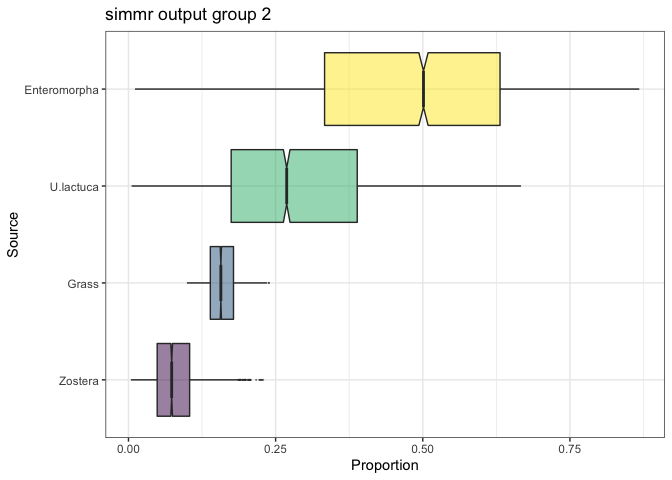``````plot(simmr_groups_out,
type = c('density','matrix'),
group = 6,
title = 'simmr output group 6')``````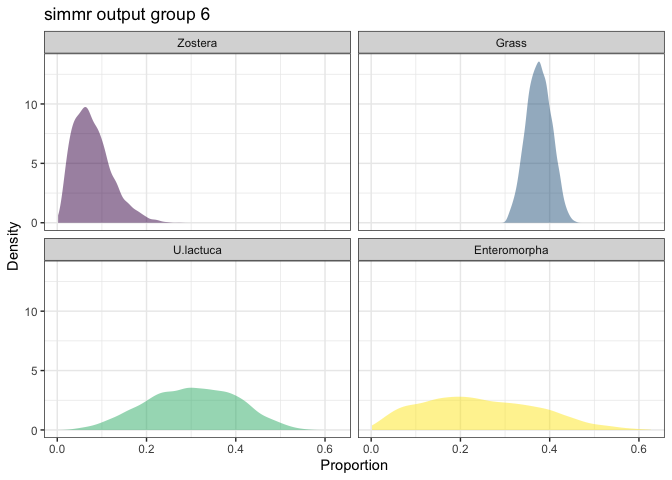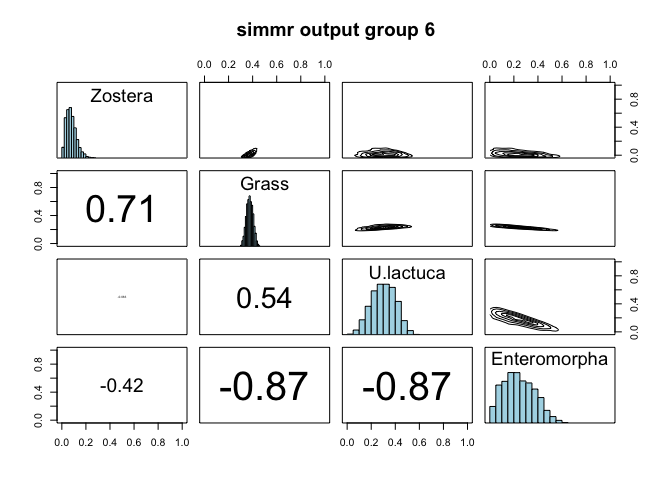Whilst you can use the `compare_sources` function for multi-group data, there is also an extra function for comparing a single source between groups via the `compare_groups` function. This allows for probabilistic output and plots comparing a single source across different groups. The simplest use is where you want to compare just two groups:

``````compare_groups(simmr_groups_out,
source = 'Zostera',
groups = 1:2)``````
``## Prob ( proportion of Zostera in group period 1 > proportion of Zostera in group period 2 ) = 1``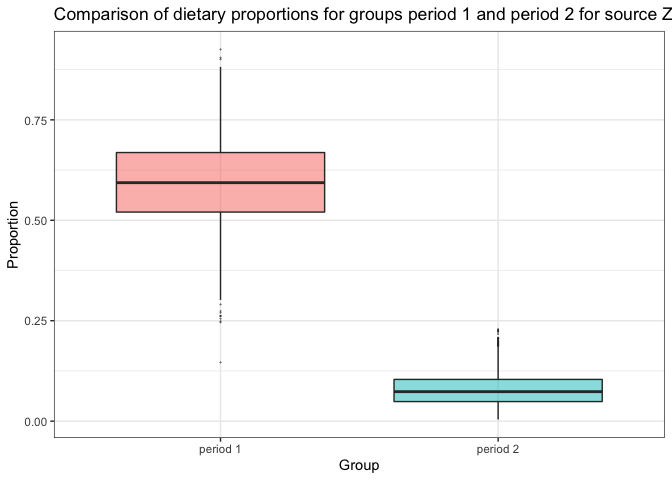This produces the probability of the group 1 dietary proportion of Zostera being greater than that of group 2. It also produces a boxplot of the difference between the dietary proportions and will save this into a new object if specified. You can turn the plot off by adding the argument `plot = FALSE`.

If you specify more than two groups `simmr` will produce the most likely probabilistic orderings of the groups as well as the boxplot as before:

``````compare_groups(simmr_groups_out,
source = 'Zostera',
groups = 1:3)``````
``````## Most popular orderings are as follows:
##                                Probability
## period 1 > period 2 > period 3      0.8186
## period 1 > period 3 > period 2      0.1814``````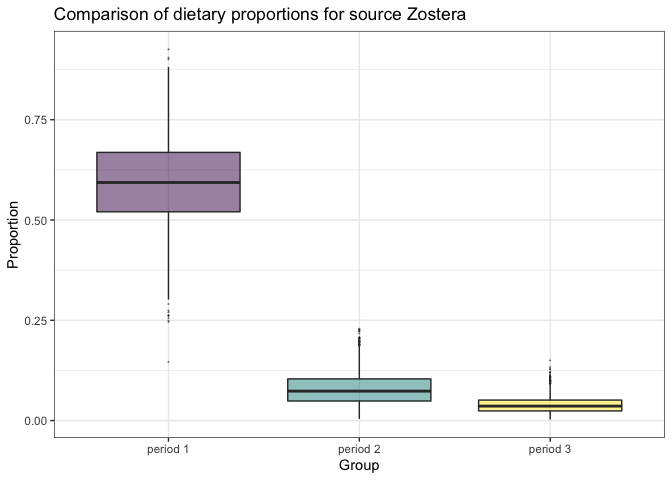## Combining sources

A common request is that of combining sources. We would recommend always doing this after running simmr, known as a-posteriori combining. Suppose for example, you wish to combine the U.lactuca and Enteromorpha sources which lie in a similar region in the isospace plot of the Geese data. To proceed, we can create a new `simmr` object using the `combine_sources` function:

``````simmr_out_combine = combine_sources(simmr_out,
to_combine = c('U.lactuca',
'Enteromorpha'),
new_source_name = 'U.lac+Ent')
plot(simmr_out_combine\$input)``````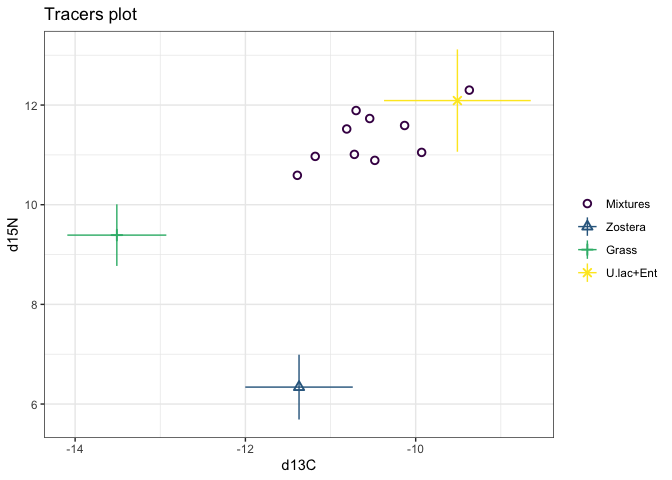``````plot(simmr_out_combine,
type = 'boxplot',
title = 'simmr output: combined sources')``````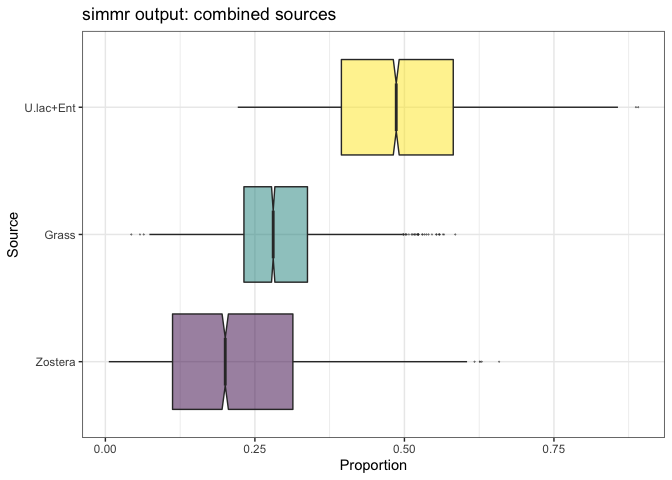This will also work with multiple groups:

``````simmr_groups_out_combine = combine_sources(simmr_groups_out,
to_combine = c('U.lactuca',
'Enteromorpha'),
new_source_name = 'U.Lac+Ent')
plot(simmr_groups_out_combine\$input,
group = 1:8)``````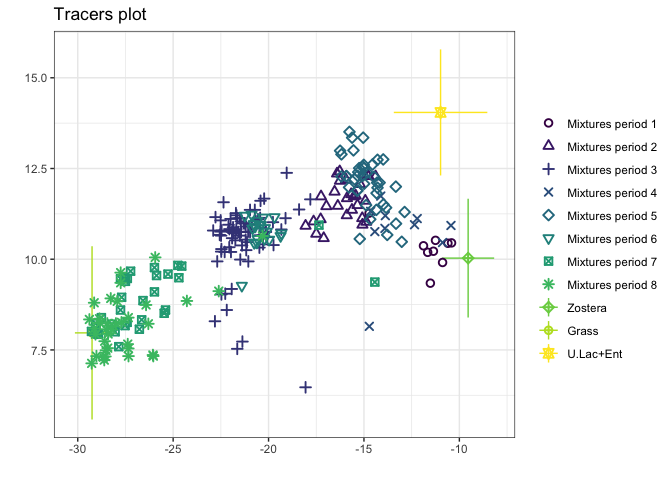``````plot(simmr_groups_out_combine,
type = 'boxplot',
title = 'simmr output: combined sources',
group = 8)``````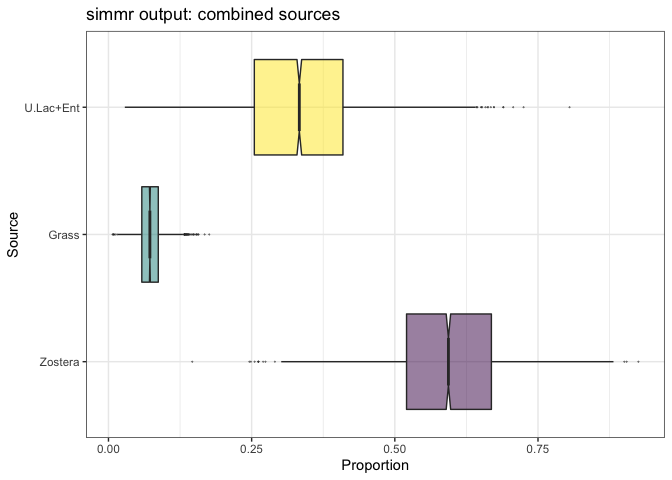``````plot(simmr_groups_out_combine,
type = 'matrix',
title = 'simmr output: combined sources',
group = 8)``````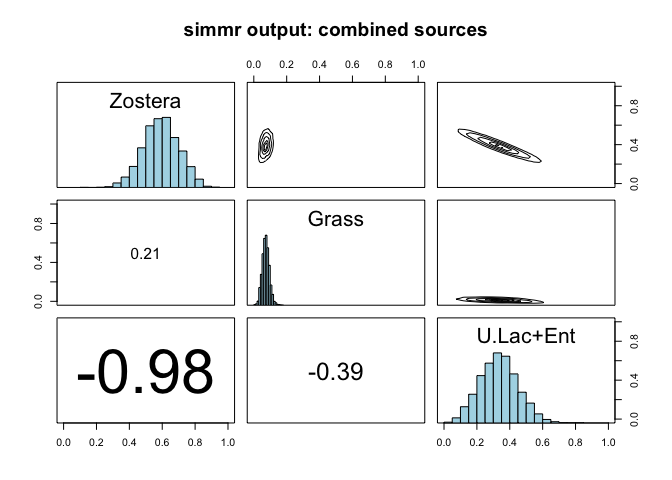``````# And we can now compare sources across groups on this new data set
compare_groups(simmr_groups_out_combine,
source = 'U.Lac+Ent',
group = 1:3)``````
``````## Most popular orderings are as follows:
##                                Probability
## period 2 > period 3 > period 1      0.9536
## period 2 > period 1 > period 3      0.0450
## period 3 > period 2 > period 1      0.0008
## period 1 > period 2 > period 3      0.0006``````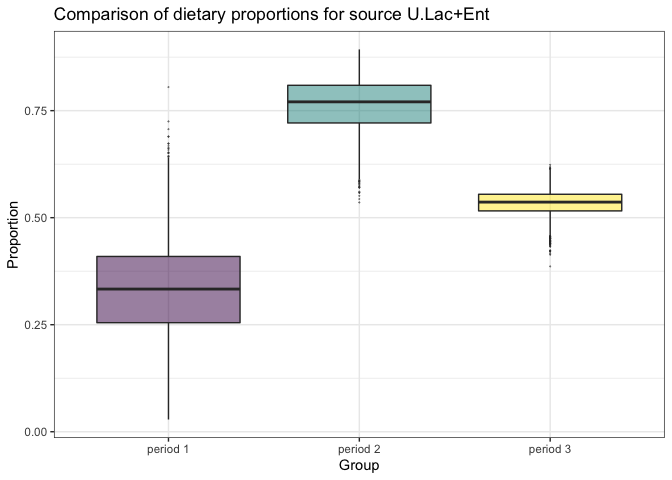## Running simmr with only one isotope

`simmr` will run fine with only one tracer, and no changes should be required to any of the functions. Here is an example with only one isotope:

``````mix = matrix(c(-10.13, -10.72, -11.39, -11.18, -10.81, -10.7, -10.54,
-10.48, -9.93, -9.37), ncol = 1, nrow = 10)
colnames(mix) = c('d13C')
s_names = c("Zostera", "Grass", "U.lactuca", "Enteromorpha")
s_means = matrix(c(-14, -15.1, -11.03, -14.44), ncol = 1, nrow = 4)
s_sds = matrix(c(0.48, 0.38, 0.48, 0.43), ncol = 1, nrow = 4)
c_means = matrix(c(2.63, 1.59, 3.41, 3.04), ncol = 1, nrow = 4)
c_sds = matrix(c(0.41, 0.44, 0.34, 0.46), ncol = 1, nrow = 4)
conc = matrix(c(0.02, 0.1, 0.12, 0.04), ncol = 1, nrow = 4)``````

Now load in with `simmr_load`:

``````simmr_in_1D = simmr_load(mixtures = mix,
source_names = s_names,
source_means = s_means,
source_sds = s_sds,
correction_means = c_means,
correction_sds = c_sds,
concentration_means =   conc)``````

Create a plot. `plot.simmr_input` automatically creates a 1D version of these plots:

``plot(simmr_in_1D)``